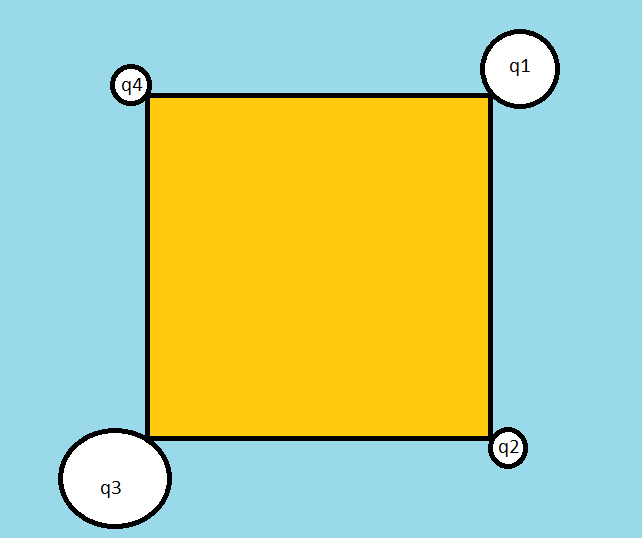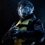# Is it possible to solve thisSuppose i have the following system of 4 different charges and different masses arranged at the corner of the square and i know the initial electrostatic energy of the system, and i want to know the speeds of individual charges when the system expands due to electrostatic repulsion uptill infinity (all charges free to move)

Is that possible? i feel that energy and momentum conservation are just not giving enough equations,, and even taking angular momentum about com does'nt seem to make it any easier either,,, infact what i basically fail to understand is how to find in what ratio the net electrostatic energy of the system is distributed among them as they move to infinity,

It is easier if they were like charges.,, which is a common question, but this seems harder,, impossible,,

But nothings supposed to have an infinite number of solutions in physics,, there should be a definite speeds with which each particle should end up with,, what am i missing?

I request any one with the knowledge i seek to enlighten me regarding this with proper explanation hopefully through some math.Note by Mvs Saketh
6 years, 9 months ago

This discussion board is a place to discuss our Daily Challenges and the math and science related to those challenges. Explanations are more than just a solution — they should explain the steps and thinking strategies that you used to obtain the solution. Comments should further the discussion of math and science.

When posting on Brilliant:

• Use the emojis to react to an explanation, whether you're congratulating a job well done , or just really confused .
• Ask specific questions about the challenge or the steps in somebody's explanation. Well-posed questions can add a lot to the discussion, but posting "I don't understand!" doesn't help anyone.
• Try to contribute something new to the discussion, whether it is an extension, generalization or other idea related to the challenge.

MarkdownAppears as
*italics* or _italics_ italics
**bold** or __bold__ bold
- bulleted- list
• bulleted
• list
1. numbered2. list
1. numbered
2. list
Note: you must add a full line of space before and after lists for them to show up correctly
paragraph 1paragraph 2

paragraph 1

paragraph 2

[example link](https://brilliant.org)example link
> This is a quote
This is a quote
    # I indented these lines
# 4 spaces, and now they show
# up as a code block.

print "hello world"
# I indented these lines
# 4 spaces, and now they show
# up as a code block.

print "hello world"
MathAppears as
Remember to wrap math in $$ ... $$ or $ ... $ to ensure proper formatting.
2 \times 3 $2 \times 3$
2^{34} $2^{34}$
a_{i-1} $a_{i-1}$
\frac{2}{3} $\frac{2}{3}$
\sqrt{2} $\sqrt{2}$
\sum_{i=1}^3 $\sum_{i=1}^3$
\sin \theta $\sin \theta$
\boxed{123} $\boxed{123}$

Sort by:

Which book do you practice physics problems from?Any advanced book?

- 6 years, 9 months ago

No advanced book, i think that if properly done, DC pandey , HC verma and iridov are just enough,, after that it depends on the person as to how creative he/she can be when solving physics problems, Brilliant is really helpful,, i just randomnly collect problems wherever available,

- 6 years, 9 months ago

Hey saketh I think here we need the concept of reduced mass. I have feeling about that but Since I didn't study this concept yet so can't able to help you. May be I'am wrong but i just share my views.

- 6 years, 9 months ago

It is a numerically indeterminate problem.You can write the acceleration of each particle as a function of the distance between the other four particles. And we will get a set of second order differential equations which cannot be solved. But i am sure if you enter this system in a computer simulation you will get a solution for the velocities. I had a similar doubt . Consider a rod resting on a rough wall and a rough floor in equilibrium at some given angle. you cannot determine the friction normal , etc. anywhere because you dont have sufficient equations.

- 6 years, 7 months ago